# Google Code Jam Archive — Round 1B 2021 problems

## Overview

Unusually for Code Jam, Round 1B happened on Sunday in a large part of the world, and even early Monday in the eastern-most timezones. To make the timing even more strange, we started off with a Broken Clock that required quite a bit of math to use it to tell the time properly. It was a particularly challenging first problem for a Round 1. Next up was the tricky Subtransmutation, which required some combinatorial insights but could be solved quickly by following some mathematical intuition and experimentation. The last problem was the interactive Digit Blocks, that could be approached in multiple ways.

Because of the high difficulty floor of this round's problems, the first submission that solved a full problem did not happen until after the 10 minute mark and less than 5000 coders managed to get some points out of more than 6600 that submitted. The first perfect score took 44 minutes, and gave neal_wu the first place in the round. zscoder and Egor were the only others to get a perfect score with less than an hour of penalty, rounding out the top 3. Ultimately, 183 coders managed a perfect score. The unofficial cutoff is 31 points, which corresponds to solving Subtransmutation. There were a lot of combinations of partial solutions that could get you over that score and into Round 2.

As usual, the Code Jam team will spend a few days finalizing the results. You can have fun reading the analyses in the problem pages while you wait. Congratulations to all advancers, and for everyone else, there is one more chance to advance to Round 2 in less than a week. Check the schedule and don't miss Round 1C next weekend!

Cast

Broken Clock: Written by Pablo Heiber. Prepared by Artem Iglikov.

Subtransmutation: Written and prepared by Yossi Matsumoto.

Digit Blocks: Written by Pablo Heiber. Prepared by Petr Mitrichev.

Solutions and other problem preparation and review by Artem Iglikov, Bir Bahadur Khatri, Darcy Best, Hannah Angara, Ikumi Hide, Jing Wang, Kevin Tran, Liang Bai, Max Ward, Md Mahbubul Hasan, Mohamed Yosri Ahmed, Nafis Sadique, Pablo Heiber, Petr Mitrichev, Pi-Hsun Shih, Timothy Buzzelli, and Yossi Matsumoto.

All analyses written by Pablo Heiber.

## A. Broken Clock

### Problem

Emmett found an old clock in his attic. The clock is a circle with 3 hands that attach to the center and rotate clockwise at constant speeds. They are called the hours hand, the minutes hand and the seconds hand. At midnight, all hands point up. The hours hand completes a full revolution in $$12$$$hours, the minutes hand completes a full revolution in $$1$$$ hour, and the seconds hand completes a full revolution in $$1$$$minute. $$1$$$ hour is equal to $$60$$$minutes, $$1$$$ minute is equal to $$60$$$seconds, and $$1$$$ second is equal to $$10^9$$$nanoseconds. For example, the clock depicted below is showing that the time is exactly $$6$$$ hours and $$30$$$minutes after midnight. The hours hand (short black) is halfway between $$6$$$ and $$7$$$(completed $$6.5/12$$$ of a revolution), the minutes hand (long black) is pointing straight down because it has completed exactly $$6$$$and a half full revolutions and the seconds hand (red) is pointing straight up because it has completed an integer number of full revolutions.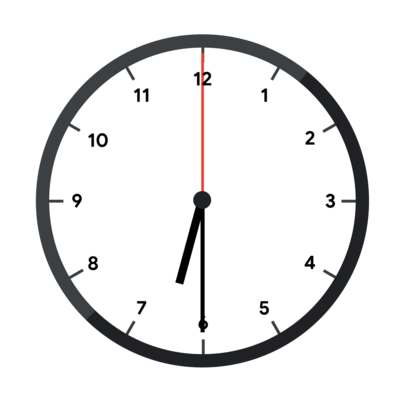Unfortunately, the hands are broken, so they all look identical and there is no way to know which hand is which. The clock in the picture above, with its hands broken, would look like this.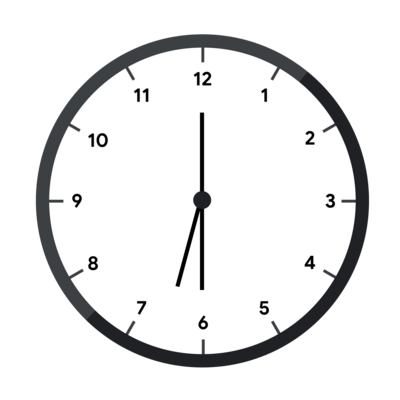In addition, no markings remain that allow Emmett to know which way is up, so any rotation of the clock could be the correct one (the clock can only be rotated, not reflected). To continue with our example, the fully broken clock could look like this.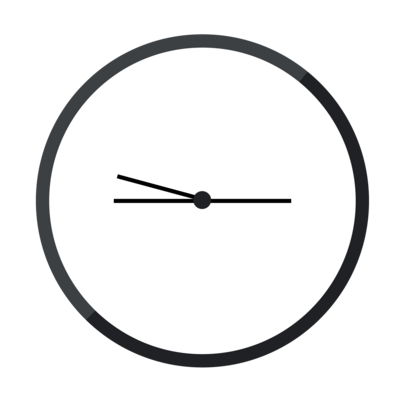Emmett does know that the time was strictly before noon, that is, strictly less than $$12$$$ hours after midnight. Emmett has taken a picture of the clock. Given that picture (represented by the angles of the hands relative to a single arbitrary axis), figure out what time it could correspond to.

Notice that Emmett has already figured out a viable orientation of the clock in some cases (Test Set 1) and has managed to narrow down the possible times to a whole integer number of seconds (Test Sets 1 and 2) or nanoseconds (Test Set 3). Please see the limits sections for more details.

### Input

The first line of the input gives the number of test cases, $$\mathbf{T}$$$. $$\mathbf{T}$$$ lines follow. Each line describes a test case and contains three integers $$\mathbf{A}$$$, $$\mathbf{B}$$$, and $$\mathbf{C}$$$: the angles of each hand, relative to an arbitrary axis and given in ticks in the clockwise direction. $$1$$$ tick is equal to $$1/12 \times 10^{-10}$$$degrees. This means that the hours hand rotates exactly $$1$$$ tick each nanosecond, the minutes hand rotates exactly $$12$$$ticks each nanosecond and the seconds hand rotates exactly $$720$$$ ticks each nanosecond.

### Output

For each test case, output one line containing Case #$$x$$$: $$h$$$ $$m$$$$$s$$$ $$n$$$, where $$x$$$ is the test case number (starting from 1) and $$h$$$, $$m$$$, $$s$$$, and $$n$$$ are integers: $$h$$$is the number of full hours since midnight (between $$0$$$ and $$11$$$, inclusive), $$m$$$ is the number of full minutes since the last full hour (between $$0$$$and $$59$$$, inclusive), $$s$$$is the number of full seconds since the last full minute (between $$0$$$ and $$59$$$, inclusive) and $$n$$$ is the number of full nanoseconds since the last full second (between $$0$$$and $$10^9-1$$$, inclusive).

### Limits

Time limit: 30 seconds.
Memory limit: 1 GB.
$$1 \le \mathbf{T} \le 100$$$. $$0 \le \mathbf{A} \le \mathbf{B} \le \mathbf{C} \lt 360 \times 12 \times 10^{10}$$$.

#### Test Set 1 (Visible Verdict)

There is a time $$t$$$that corresponds to the input such that: • $$t$$$ is an integer number of seconds after midnight.
• $$t$$$can be read from the input clock without rotating it. #### Test Set 2 (Visible Verdict) There is a time that corresponds to the input and is an integer number of seconds after midnight. #### Test Set 3 (Visible Verdict) There is a time that corresponds to the input and is an integer number of nanoseconds after midnight. ### Sample Note: there are additional samples that are not run on submissions down below. Sample Input 3 0 0 0 0 21600000000000 23400000000000 1476000000000 2160000000000 3723000000000  Sample Output Case #1: 0 0 0 0 Case #2: 6 30 0 0 Case #3: 1 2 3 0  In Sample Case #1, all hands point up (as in the first picture below) which happens only exactly at midnight (as in the second picture below).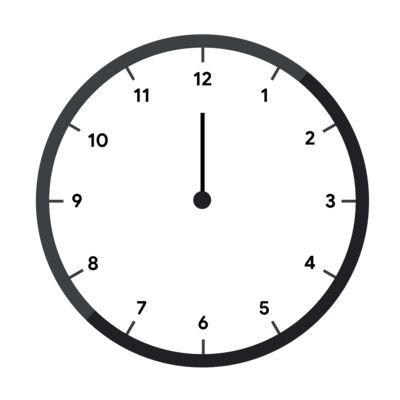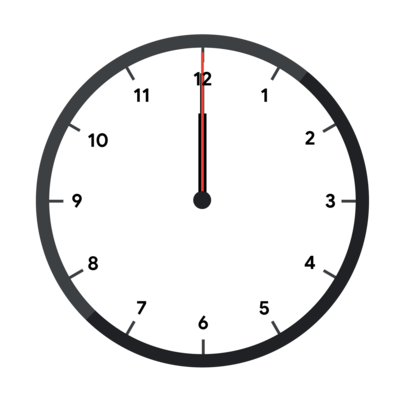Sample Case #2 is the one pictured in the main part of the statement. The angles of the hands in degrees are $$0$$$, $$180$$$and $$195$$$. These angles can correspond to $$6$$$⁠h $$30$$$⁠m $$0$$$⁠s without rotating the clock, as the pictures in the main part of the statement show. Notice however, that at $$0$$$⁠h $$30$$$⁠m $$0$$$⁠s (pictured below), the clock looks the same but rotated 180 degrees.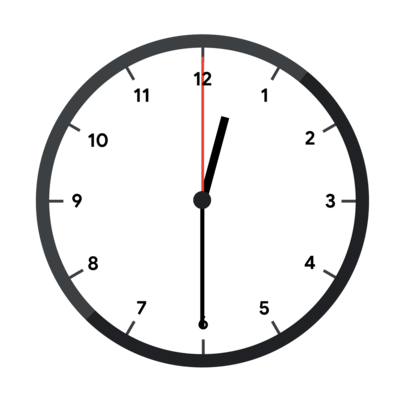Even in Test Set 1, $$0$$$⁠h $$30$$$⁠m $$0$$$⁠s would be a valid answer. The limit only says that there is one valid time that does not require rotating the clock, but times that work with rotation are also valid answers. In Sample Case #3, the input represents the clock in the first picture below and the given output happens when interpreting the clock as in the second picture below.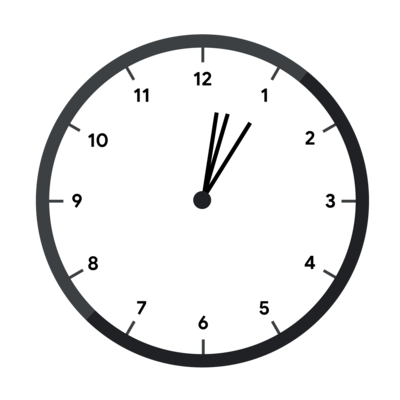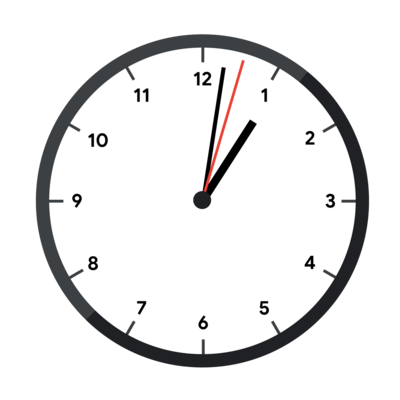### Additional Sample - Test Set 2 The following additional sample fits the limits of Test Set 2. It will not be run against your submitted solutions. Sample Input 3 5400000000000 5400000000000 5400000000000 10800000000000 32400000000000 34200000000000 23076000000000 23760000000000 25323000000000  Sample Output Case #1: 0 0 0 0 Case #2: 0 30 0 0 Case #3: 1 2 3 0  Sample Cases in this test set are the same as in the previous one, but the clock is rotated by $$45$$$, $$90$$$, and $$180$$$ degrees clockwise respectively, as shown below.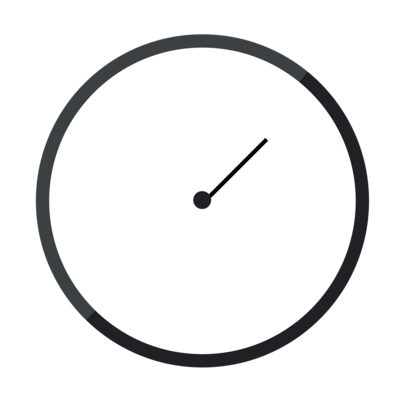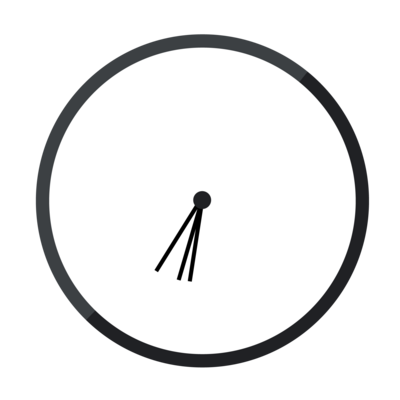### Additional Sample - Test Set 3

The following additional sample fits the limits of Test Set 3. It will not be run against your submitted solutions.
Sample Input
1
0 11 719

Sample Output
Case #1: 0 0 0 1


### Limits

Time limit: 30 seconds.
Memory limit: 1 GB.
$$1 \le \mathbf{T} \le 100$$$. $$1 \le \mathbf{N} \le 20$$$.
$$0 \le \mathbf{U_i} \le 20$$$, for all $$i$$$.
$$1 \le \mathbf{U_N}$$$. $$2 \le \mathbf{U_1} + \mathbf{U_2} + \cdots + \mathbf{U_N}$$$.

#### Test Set 1 (Visible Verdict)

$$\mathbf{A} = 1$$$. $$\mathbf{B} = 2$$$.

$$1 \le \mathbf{A} \lt \mathbf{B} \le 20$$$. ### Sample Note: there are additional samples that are not run on submissions down below. Sample Input 3 2 1 2 1 2 5 1 2 2 0 0 0 1 3 1 2 1 1 1  Sample Output Case #1: 4 Case #2: 6 Case #3: 5  In Sample Case #1, we require one unit of metal $$1$$$ and two units of metal $$2$$$. If we start with a single unit of metal $$3$$$, then applying the spell once will give us one unit of metal $$1$$$and one unit of metal $$2$$$. There is no way to get an additional unit of metal $$2$$$. Similarly, starting with a single unit of metals $$1$$$ or $$2$$$is not sufficient. However, a single unit of metal $$4$$$ is sufficient as is demonstrated in the picture in the main part of the statement.

In Sample Case #2, we can start with a single unit of metal $$6$$$and apply the following operations: • Apply spell on $$6$$$: $$\{6\} \longrightarrow \{4, 5\}$$$. • Apply spell on $$4$$$: $$\{4, 5\} \longrightarrow \{2, 3, 5\}$$$. • Apply spell on $$2$$$: $$\{2, 3, 5\} \longrightarrow \{1, 3, 5\}$$$. • Apply spell on $$3$$$: $$\{1, 3, 5\} \longrightarrow \{1, 1, 2, 5\}$$$. Note that even though we have an extra unit of metal $$2$$$, this solution is valid.

In Sample Case #3, we can start with a single unit of metal $$5$$$and apply the following operations: • Apply spell on $$5$$$: $$\{5\} \longrightarrow \{3, 4\}$$$. • Apply spell on $$4$$$: $$\{3, 4\} \longrightarrow \{2, 3, 3\}$$$. • Apply spell on $$2$$$: $$\{2, 3, 3\} \longrightarrow \{1, 3, 3\}$$$. • Apply spell on $$3$$$: $$\{1, 3, 3\} \longrightarrow \{1, 1, 2, 3\}$$$. There are other ways to apply spells which also work but they all require starting with a single unit of metal $$5$$$ or higher.

### Additional Sample - Test Set 2

The following additional sample fits the limits of Test Set 2. It will not be run against your submitted solutions.
Sample Input
3
3 2 4
1 1 1
3 2 4
1 0 1
5 2 5
1 0 0 0 1

Sample Output
Case #1: IMPOSSIBLE
Case #2: 5
Case #3: 10


If the judge receives an invalidly formatted line, an invalid tower number, or the number of a tower that already contains $$\mathbf{B}$$$blocks from your program, the judge will print a single number -1. After the judge prints -1 for any of the reasons explained above, it will not print any further output. If your program continues to wait for the judge after receiving a -1, your program will time out, resulting in a Time Limit Exceeded error. Notice that it is your responsibility to have your program exit in time to receive a Wrong Answer judgment instead of a Time Limit Exceeded error. As usual, if the memory limit is exceeded, or your program gets a runtime error, you will receive the appropriate judgment. You can assume that the digit for each block is generated uniformly at random, and independently for each digit, for each test case and for each submission. Therefore even if you submit exactly the same code twice, the judge could use different random digits. ### Limits Time limit: 60 seconds. Memory limit: 1 GB. $$\mathbf{T} = 50$$$.
$$\mathbf{N} = 20$$$. $$\mathbf{B} = 15$$$.

#### Test Set 2 (Visible Verdict)

$$\mathbf{P} = 937467793908762347$$$(approximately $$9.37 \times 10^{17}$$$).
Note that this boundary is chosen as approximately $$98\%$$$of $$\mathbf{T} \times S$$$.

### Testing Tool

You can use this testing tool to test locally or on our platform. To test locally, you will need to run the tool in parallel with your code; you can use our interactive runner for that. For more information, read the instructions in comments in that file, and also check out the Interactive Problems section of the FAQ.

Instructions for the testing tool are included in comments within the tool. We encourage you to add your own test cases. Please be advised that although the testing tool is intended to simulate the judging system, it is NOT the real judging system and might behave differently. If your code passes the testing tool but fails the real judge, please check the Coding section of the FAQ to make sure that you are using the same compiler as us.

Sample Interaction
Judge
Solution
2 3 3 1500

Judge provides $$\mathbf{T}$$$, $$\mathbf{N}$$$, $$\mathbf{B}$$$, $$\mathbf{P}$$$
3

Judge provides a block with a 3 on it
1

Solution places it on pile 1
2

Judge provides a block with a 2 on it
1

Solution places it on pile 1
5

2

4

2

1

1

We are now at the state shown in Picture 1
6

3

3

2

9

3

0

3

We are now at the state shown in Picture 4 (sum = 564)
7

Judge provides the first block in the second case
3

Solution places it on pile 3
8

Judge provides the final block in the second case
2

Solution places it on pile 2 (sum = 1285)
1

Judge confirms that the sum of both test cases (1849) is at least 1500

## Analysis — A. Broken Clock

### Test Set 1

In Test Set 1, our only unknown is which hand is which. Since there are only $$3!$$$possibilities for the assignment of angles in the input to a specific hand, we can just try them all. After we have angles assigned to specific hands, we can just read the time. The easiest way is to just check the hours hand, as the statement tells us it moves one tick per nanosecond, so the current number of nanoseconds after midnight is equal to the angle in ticks of the hours hand. Notice that we still need to check that the time in the other two hands is consistent with this! We can do this by reading the number of minutes and seconds from their hands using the definitions in the statements, and see if it is consistent with the current number of minutes and seconds as computed from the nanoseconds we got from the hours hand. Alternatively, we can do the reverse process of getting from current time to how the clock should look. We do that in more detail below, as it is needed to solve the other test sets. The solution to Test Sets 2 and 3 is based on first solving the inverse problem: given a time of the day, find a canonical set of $$3$$$ angles that would produce it. Luckily, the way to solve it is explained on the statement: we know the speeds of each hand so we can translate a time of the day into a number of ticks since midnight, and then take it modulo the number of ticks in a full circle ($$12 \times 10^{10} \times 360$$$). Once we get three angles, we need to consider the potential rotations. We can say that canonical sets of angles always have at least one $$0$$$, but there can be up to $$3$$$ways to do that (choosing each hand to be the $$0$$$ one): simply choosing the lexicographically least of them (after sorting them) suffices.

In Test Set 2, there are only $$12 \times 60 \times 60$$$possible times of the day to consider. We can simply build the canonical representation $$a$$$ for each time $$t$$$and store it in a reverse dictionary from representations to times (which one we save if there are multiples does not matter) as a first step. Then, for each input, we get its canonical representation and find a corresponding time in the dictionary to output. ### Test Set 3 In Test Set 3, the number of different times of the day is too large to check them all individually. A possible approach is to restrict the number of times of the day that can correspond to a specific input set of angles $$a$$$ and then check each of those to see if anyone fully matches by using the reverse conversion.

We can try every possible assignment of each angle to a specific hand. There are only $$3!$$$possibilities for that. Let us say that we now have an angle of $$a_h$$$ for the hours hand, $$a_m$$$of the minutes hand and $$a_s$$$ for the seconds hand.

Let us write the real time as $$h$$$full hours plus $$n$$$ nanoseconds, that is, $$h \cdot 3600 \cdot 10^9 + n$$$nanoseconds since midnight, with $$h$$$ between $$0$$$and $$11$$$. The angle (in ticks) of the minute hand is $$12 \cdot n$$$and the angle of the hour hand is $$h \cdot 3600 \cdot 10^9 + n$$$. The difference between those two numbers $$h \cdot 3600 \cdot 10^9 - 11 \cdot n$$$needs to be equal to the difference between the angles we read from the input $$a_h - a_m$$$. Note that this works because the difference between angles is invariant through rotations. If we try every possible value for $$h$$$between $$0$$$ and $$11$$$, every variable except $$n$$$ in the last equality has an actual value, and we can solve for $$n$$$. If the value for $$n$$$ happens to be an integer, it gives us a real time candidate.

Finally, for each of the up to $$3! \cdot 12$$$real time candidates, we do the reverse check as we did in the previous solution. Test Data info We recommend that you practice debugging solutions without looking at the test data. ## Analysis — B. Subtransmutation To solve this problem, we can first tackle a simplified version: given a unit of metal $$x$$$, can it be used to produce all the metals required by the input?

To solve this simplified problem, we can use a greedy strategy. Keep track of the multiset $$H$$$of units of metal we have (starting with $$\{x\}$$$) and the multiset $$D$$$of units of metal we owe (starting with the ones given as input). Iterate the following steps: 1. Remove the intersection $$H \cap D$$$ from both $$H$$$and $$D$$$.
2. If $$D$$$is empty, the answer is yes. 3. If $$H$$$ is empty and $$D$$$is not, the answer is no. 4. Take all $$c$$$ units of the metal $$i$$$with the largest number still in $$H$$$. Remove all $$c$$$units of $$i$$$ from $$H$$$and insert $$c$$$ units of $$i - \mathbf{A}$$$and $$c$$$ units of $$i - \mathbf{B}$$$into $$H$$$ (if either new metal number is invalid, skip those).

This procedure works because those units that we greedily transform are not something that we owe, so we have nothing better to do with them (it may be futile to convert them, but it does not hurt). And since we have to produce the units that we owe, we might as well just pay them back as soon as possible, as anything we could do with the current unit we could also do with a future unit that we would use to pay the debt instead.

In Test Set 1, notice that if it is possible to find an answer $$m$$$for an input where $$\mathbf{N}$$$ and all $$\mathbf{U_i}$$$have maximum value, that procedure also produces enough units for any other possible input. If we implement the greedy strategy above and try it for this particular input on increasingly large values for $$m$$$, we can quickly arrive at the realization that $$m=29$$$solves it. This means that all inputs can be generated with a metal with number no greater than $$29$$$. So, using the same procedure of checking increasingly large values of $$m$$$for the given input is a valid solution for the full Test Set. It is also possible to notice and/or prove theoretically that there are no impossible cases and that the answers are small. A quick argument is that numbers grow exponentially: from a single unit of number $$m$$$ we can create 2 units of number $$m-2$$$(with leftovers). This means we can create $$2^i$$$ units of element $$m-2i$$$from a single unit of element $$m$$$. We can distribute these among the elements $$m-2i-\mathbf{N}+1$$$through $$m-2i$$$ (with a lot of leftovers), getting $$2^i / \mathbf{N}$$$units of each of $$\mathbf{N}$$$ consecutive elements. These can be further moved to elements with lower numbers if needed. This shows that an $$m$$$such that $$2^{m - 2 \times 20} / 20 \gt 20$$$ ought to be enough, and $$m=49$$$fulfills such a requirement. ### Test Set 2 As the samples show, impossible cases can happen in Test Set 2. This means running our Test Set 1 solution as is will result in the program not finishing and getting a Time Limit Exceeded error. A simple solution, however, is to notice that there is also a somewhat small maximum answer for all possible cases, and then simply stop once we are past it and return impossible. Knowing what that maximum answer is, and proving it, is harder as we cannot just rely on experiments. We have to lean more on the theory of the problem. We can first notice that starting from metal $$m$$$, every metal we can possibly produce has the same remainder as $$m$$$modulo the greatest common divisor between $$\mathbf{A}$$$ and $$\mathbf{B}$$$(let us call that $$g$$$). This is an application of Diophantine equations, but while knowing that specific theory could help tackle the problem, it is not absolutely necessary. Now, consider the remainder modulo $$g$$$of every $$i$$$ such that $$\mathbf{U_i} \gt 0$$$. If they are all the same value $$k$$$, then $$m$$$modulo $$g$$$ needs to be $$k$$$as well. If they are not all the same, then the case is impossible. At this point, we can express all metal numbers that matter as $$g \times x + k$$$ for some $$x$$$. To show that all of remaining cases are possible, we can generalize the proof we did for Test Set 1 and use Bézout's identity to build them, or we can simply iterate all relatively prime values for $$\mathbf{A} / g$$$ and $$\mathbf{B} / g$$$and solve the largest case experimentally. If we do that, we can arrive at the conclusion that the maximum possible answer for a possible Test Set 2 case is $$402$$$. Therefore, we can do the same solution as in Test Set 1, exploring values for $$m$$$up to $$402$$$, and returning impossible if we did not find an answer.

Test Data
info We recommend that you practice debugging solutions without looking at the test data.

## Analysis — C. Digit Blocks

Let $$D_{i,j}$$$be the digit on the block that has exactly $$j$$$ blocks under it in the $$i$$$-th tower. The number written on the $$i$$$-th tower is then \sum_{j=0}^{\mathbf{B}-1} 10^j \cdot D_{i,j} and the total score is \sum_{i=1}^{\mathbf{N}} \sum_{j=0}^{\mathbf{B}-1} 10^j \cdot D_{i,j}.

Notice that each term in the last sum is independent from others, that is, each position in a tower has a particular value that gets multiplied by the digits, and exchanging blocks at the same heights that go on different towers does not change the final outcome. Our job to maximize the score is to get the largest digits paired up with the largest values. That means, putting large digits near the top of the towers. Moreover, the value of a position is more than $$9$$$times the value of all the positions below it combined. Therefore, delaying the use of a position until we can get the maximum possible digit can be worth sacrificing the ability to decide on the order of all positions below it for up to $$9$$$ towers, and possibly a lot more (as even a random order gets some value).

All the approaches we present are based on trying to reserve high-valued positions for high-valued digits, sacrificing other positions.

### Greedy strategies

The simplest greedy strategy is: try to place $$9$$$⁠s in the top position of every tower, and sacrifice everything else. That means, when a block comes, if it is a $$9$$$, place it in the highest non-finished tower we have. If it is not, place it in the highest tower we have that has fewer than $$\mathbf{B}-1$$$blocks on it. This reserves the top spots for $$9$$$⁠s and accelerates the availability of newer top spots by trying to build towers up as soon as possible.

There are other similar strategies, like doing the same but trying to place $$9$$$⁠s in the top two spots of every tower, possibly pivoting to just the top spot when we are running out of time. Similarly, we can pivot to accepting lower digits for second-from-the-top spots or for top spots when there are few blocks left and the likelihood of enough $$9$$$⁠s coming becomes low.

These simple strategies oscillate between getting a score of $$87\%$$$and $$91\%$$$ of $$S$$$, which means many of them are enough to pass Test Set 1, but none seems to be close to pass Test Set 2. More sophisticated heuristics can pass Test Set 2, but they need to consider at least the top $$2$$$ positions and also deal with tactically placing not just $$9$$$⁠s but also $$8$$$⁠s. These type of solution need careful tweaking and also comes with some uncertainty that it will eventually work out. The solutions in the next section address both those issues, ensuring the points and quite possibly saving us time.

### Dynamic programming strategies

An alternative way is to try to maximize the expected score. This does not exactly maximize the probability of getting above a specific threshold (being more aggressive or conservative near the end depending on how close you actually are to the threshold might be better), but it's really close and much simpler.

The optimal such solution (the one that gets $$S$$$as its expected score) is too slow, but it is worthwhile to consider it as a starting point. By linearity of expectation, when considering where to put a block, only the heights of all current towers matters, but not the actual values that were put there (since that is the score we will get regardless of all future decisions). We can therefore consider the multiset of tower heights the status, and optimize a function f(status, next_digit) that checks every possible placement for each possible next digit. The problem with this idea is, of course, the status space is too big for the time limit. This is what we did to calculate $$S$$$, but we ran it for a lot longer than the time limit.

As we mentioned before, we can improve on the time limit by not caring too much about what happens with the low-value positions. That is, consider only a subset of the statuses. One example is this: for each digit we consider only the options of the first greedy strategy. We either put it in the top-most position of some tower, or we put it in the highest tower that has fewer than $$\mathbf{B}-1$$$blocks on it. This severely reduces the number of possible statuses, as there is only one tower that can have a height other than $$0$$$, $$\mathbf{B}-1$$$or $$\mathbf{B}$$$. Instead of making the decision greedily, we leave the decision of which option to choose to the dynamic programming part. This solution is fast enough and gets about $$96\%$$$of $$S$$$ as its score. Not enough for Test Set 2, but getting closer. Doing the same but leaving the top $$2$$$positions up for optimization, on the other hand, gets over $$99.5\%$$$ of $$S$$$and passes Test Set 2. Since the top position represents close to $$90\%$$$ of the value, it makes sense that optimizing it gets $$90\%$$$of the score. Similarly, the top two positions represent close to $$99\%$$$ of the value. More formally, consider two modified problems in which the top position or top two positions retain their value, but all other positions are valued as $$0$$$. Our proposed solutions get the maximum expected scores $$S_1$$$ and $$S_2$$$for those modified problems, and we know that $$S_1 \gt 0.9 \cdot S$$$ and $$S_2 \gt 0.99 \cdot S$$$. Therefore, our solutions' expected score is guaranteed to be above each respective threshold, and the extra score we get from the lower positions gives us an additional gap to make the probability of success extremely high: our estimates are that each solution has less than $$10^{-10}$$$ probability of failing the respective test set.